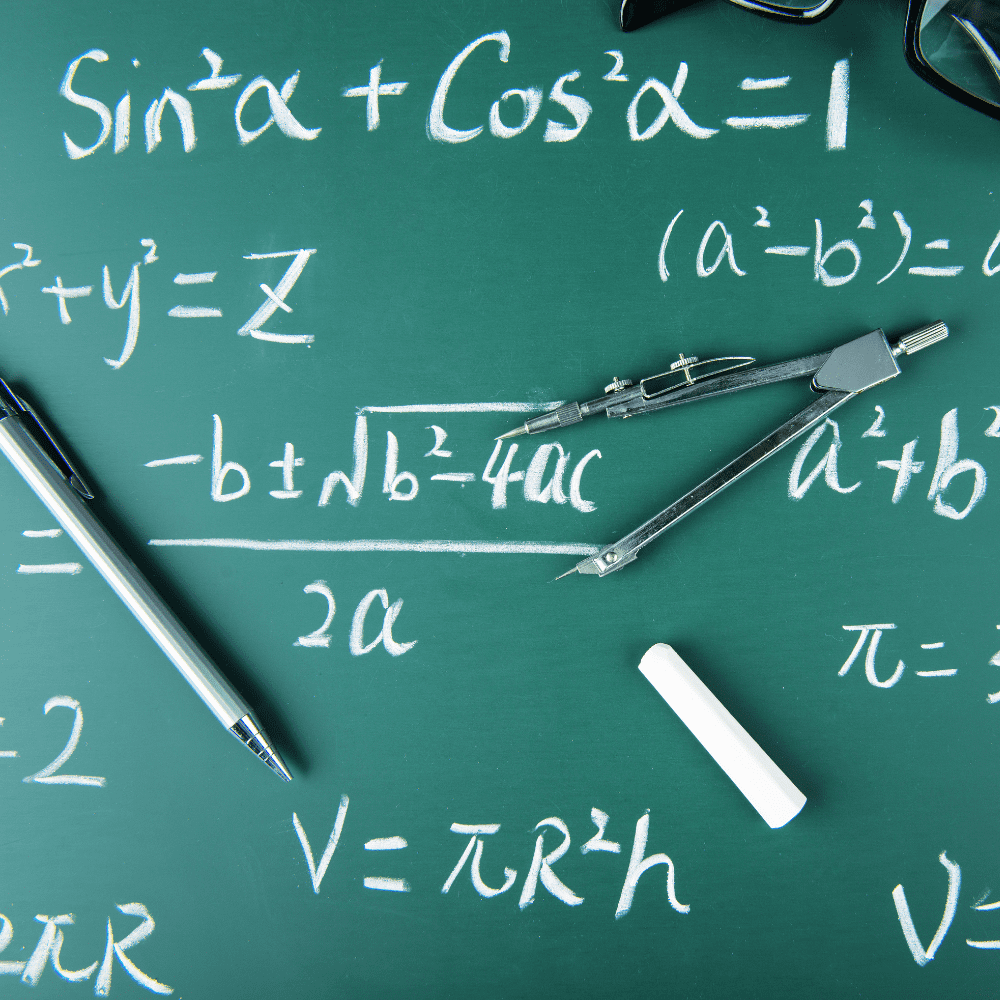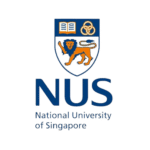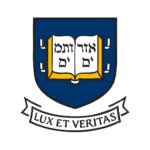# Maths Tuition

Tackle any Maths question you have and gain the skills to ace the exams
with TigerCampus Singapore’s private tuition service.## Overview

### Customised curriculum

Choose one or more subjects, and we'll find a tutor who can make sure you're prepared.

### Flexible

Take lessons only when you need them—as little or as many as necessary until you feel confident.

### Private lesson

No need to accommodate other students. Learning is customised your perfect pace and difficulty so you are always improving.

## Maths Education in Singapore

Mathematics competency is the important basis for technical and science education at the higher levels, according to the Singapore educational system.

Elementary mathematics is a required topic at the primary and secondary levels, and math tutoring professionals in Singapore may help students develop an interest in the subject from an early age.

## Description

TigerCampus Singapore is one of the premium online tuition centers in the country. We have at-home and online Maths tutors. TigerCampus tutors are able to provide quality home and online tuition to prepare you to take any maths exam. From primary level up until university, we provide tuition for any maths tuition you find necessary.

Check out our available syllabuses below and request a free trial today!

## Benefits

### Our available maths tuition syllabuses

##### Main Topics
1. Number & algebra
2. Measurement and geometry
3. Statistics
4. Whole numbers
5. Fractions
6. Money
7. Area & volume
8. Decimals
9. Data representation and interpretation
10. Data analysis
11. Percentage
12. Ratio
13. Rate & speed
##### Year 1
• Number, algebra, statistics and geometry
• Number, algebra and graphs
• Mensuration, probability and statistics
• Trigonometry, geometry and statistics
• Algebra and graphs
• Geometry and vectors

##### Year 2
• Number, algebra, graphs and geometry
• Number, probability, algebra, geometry and trigonometry
• Algebra and graphs
• Trigonometry, vectors, geometry, statistics, algebra and graph

Main Topics

1. Number & Algebra
2. Geometry & Measurement
3. Statistics & Probability
##### Main topics
1. Algebra
2. Geometry & Trigonometry
3. Calculus (Differentiation & Integration)
###### Section A: Pure Mathematics
1. Functions and Graphs
2. Exponential logarithmic functions and Graphing techniques
3. Equations and inequalities
4. Calculus
5. Differentiation
6. Integration
###### Section B: Probability and Statistics
1. Probability
2. Binomial distribution
3. Normal distribution
4. Sampling
5. Hypothesis testing
6. Correlation and Linear regression
###### Section A: Pure Mathematics
1. Algebra and Calculus
2. Mathematical Induction
3. Complex numbers
4. Polar Coordinates
5. Conic sections
6. Applications of definite integrals
7. Differential equations
8. Discrete Mathematics, Matrices and Numerical Methods
9. Recurrence relations
10. Matrices and linear spaces
11. Numerical methods
###### Section B: Probability and Statistics
1. Discrete random variables
2. Continuous random variables
3. Hypothesis testing and Confidence intervals
4. Non-parametric tests
##### Section A: Pure Mathematics
###### Algebra and Calculus
1. Mathematical induction
2. Complex numbers
3. Polar coordinates
4. Conic sections
5. Applications of definite integrals
6. Differential equations
###### Discrete Mathematics, Matrices, and Numerical Methods
1. Recurrence relations
2. Matrices and linear spaces
3. Numerical methods
##### Section B: Probability and Statistics
###### Probability and Statistics
1. Discrete random variables
2. Continuous random variables
3. Hypothesis testing and Confidence intervals
4. Non-parametric tests
##### Analysis and Approaches (SL)
1. Number & Algebra
2. Functions
3. Geometry & Trigonometry
4. Probability & Statistics
5. Calculus
6. Toolkit & Exploration

##### Applications and Interpretation (SL)
1. Number & Algebra
2. Functions
3. Geometry & Trigonometry
4. Probability & Statistics
5. Calculus
6. Toolkit & Exploration
##### Analysis and Approaches (HL)
1. Number & Algebra
2. Functions
3. Geometry & Trigonometry
4. Probability & Statistics
5. Calculus
6. Toolkit & Exploration

##### Applications and Interpretation (HL)
1. Number & Algebra
2. Functions
3. Geometry & Trigonometry
4. Probability & Statistics
5. Calculus
6. Toolkit & Exploration
##### Our tutors graduated from top universities## How it works

### 1Request a tutor

Let us know your goals and age range. We'll figure out a plan to help get you there.

### 2Match with a tutor

You'll get a tutor based on your needs and goals, or you can request a specific tutor.

### 3Start a free trial

Experience a free trial lesson with your new tutor and see if your learning style matches.

### 4Keep it up!

If everything went well, sign up to keep going! You can choose the pacing of the lessons

### 1Request a tutor

Let us know your goals and age range. We'll figure out a plan to help get you there.

### 2Match with a tutor

You'll get a tutor based on your needs and goals, or you can request a specific tutor.

### 3Start a free trial

Experience a free trial lesson with your new tutor and see if your learning style matches.

### 4Keep it up!

If everything went well, sign up to keep going! You can choose the pacing of the lessons